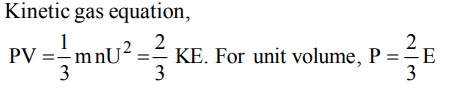## Thermodynamics Questions and Answers Part-1

1. Which of the following is closed system ?
a) Jet engine
b) Tea placed in a steel kettle
c) Pressure cooker
d) Rocket engine during propulsion

Explanation: Closed system can exchange energy and not matter with surroundings. Pressure cooker provides closed system.

2. An isolated system is that system in which:
a) There is no exchange of energy with the surroundings
b) There is exchange of mass and energy with the surroundings
c) There is no exchange of mass or energy with the surroundings
d) There is exchange of mass with the surroundings

Explanation: Isolated system cannot exchange mass or energy

3. The intensive property among these quantities is
a) mass
b) volume
c) enthalpy
d) mass/volume

Explanation: Mass/volume = density, hence it is intensive property

4. Which is an extensive property of the system ?
a) Volume
b) Viscosity
c) Temperature
d) Refractive index

Explanation: Volume depends upon mass. Hence it is extensive property

5. Internal energy and pressure of a gas of unit volume are related as :
a) $P=\frac{2}{3}E$
b) $P=\frac{1}{2}E$
c) $P=\frac{3}{2}E$
d) P = 2E

Explanation:6. The latent heat of vapourization of a liquid at 500 K and 1 atm pressure is 10.0 kcal/mol. What will be the change in internal energy $\left(\triangle U\right)$ of 3 moles of liquid at the same temperature
a) 13.0 kcal/mol
b) –13.0 kcal/mol
c) 27.0 kcal mol
d) –7.0 kcal/mol

Explanation:7. Mechanical work is specially important in systems that contain
a) solid –liquid
b) liquid –liquid
c) solid –solid
d) gases

Explanation: Gases can undergo expansion hence perform mechanical work . (P $\triangle$ V work)

8. In a closed insulated container a liquid is stirred with a paddle to increase the temperature. Which of the following is true?
a) $\triangle E=W\neq0$
b) $\triangle E=W, q = 0$
c) $\triangle E=0,W=q\neq0$
d) $W=0,\triangle W=q\neq0$

Explanation: In insulated system no heat or matter is exchanged, q = 0
$\triangle$E = W . The work done on the system increases internal energy

9. An adiabatic expansion of an ideal gas always has
a) decrease in temperature
b) q = 0
c) w = 0
d) $\triangle H=0$

10. For the reaction $C+O_{2}\rightarrow CO_{2}$
a) $\triangle H>\triangle E$
b) $\triangle H<\triangle E$
c) $\triangle H=\triangle E$